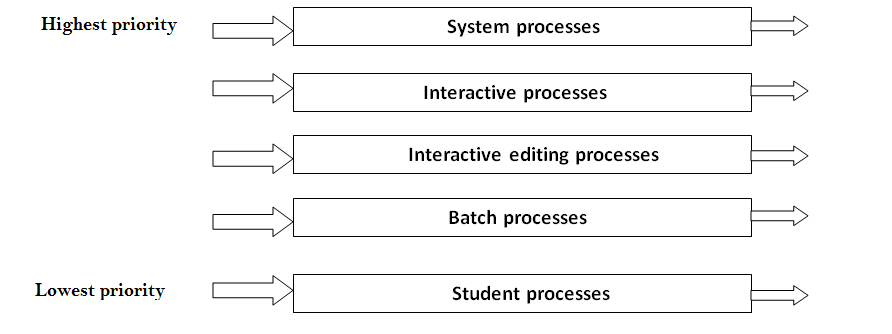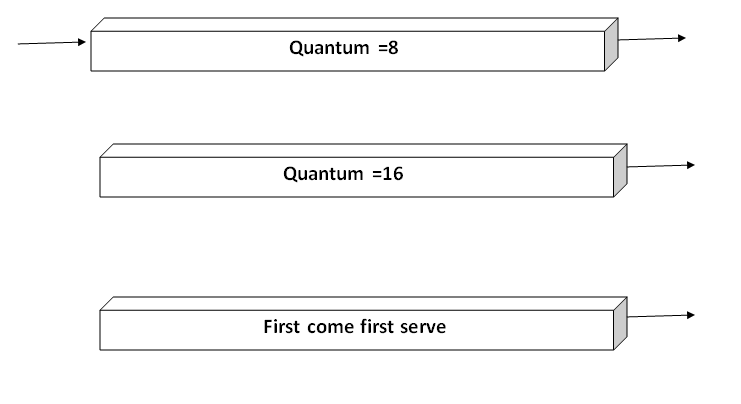# Multilevel Queue Scheduling in Operating System

In this article, we will learn about the introduction of multilevel queue scheduling and the various types of processes in multilevel queue in the operating system.
Submitted by Prerana Jain, on August 14, 2018

## Multilevel queue scheduling

Every algorithm supports a different class of process but in a generalized system, some process wants to be scheduled using a priority algorithm. While some process wants to remain in the system (interactive process) while some are background process when execution can be delayed.

In general round-robin algorithm with different time quantum is used for such maintenance. The Number of ready queue algorithm between the queue, algorithm inside a queue algo but the queues may change from system to system. There is a various class of scheduling algorithm which is created for situations like in which the processes are easily divided into the different groups. There is a common division between the foreground(interactive) process and background (batch process). There are two types processes have the different response time, the different requirement of resources so these processes need different scheduling algorithms. The foreground processes have priority(externally defined) over background processes.

In the multilevel queue scheduling algorithm partition the ready queue has divided into seven separate queues. Based on some priority of the process; like memory size, process priority, or process type these processes are permanently assigned to one queue. Each queue has its own scheduling algorithm. For example, some queues are used for the foreground process and some for the background process.

The foreground queue can be scheduled by using a round-robin algorithm while the background queue is scheduled by a first come first serve algorithm.

It is said that there will be scheduled between the queues which are easily implemented as a fixed- priority preemptive scheduling. Let us take an example of a multilevel queue scheduling algorithm with five queues:

1. System process
2. Interactive processes
3. Interactive editing processes
4. Batch processes
5. Student processes## Multilevel feedback scheduling

Generally, we see in a multilevel queue scheduling algorithm processes are permanently stored in one queue in the system and do not move between the queue. There is some separate queue for foreground or background processes but the processes do not move from one queue to another queue and these processes do not change their foreground or background nature, these type of arrangement has the advantage of low scheduling but it is inflexible in nature.

Multilevel feedback queue scheduling it allows a process to move between the queue. This the process are separate with different CPU burst time. If a process uses too much CPU time then it will be moved to the lowest priority queue. This idea leaves I/O bound and interactive processes in the higher priority queue. Similarly, the process which waits too long in a lower priority queue may be moved to a higher priority queue. This form of aging prevents starvation.

The multilevel feedback queue scheduler has the following parameters:

• The number of queues in the system.
• The scheduling algorithm for each queue in the system.
• The method used to determine when the process is upgraded to a higher-priority queue.
• The method used to determine when to demote a queue to a lower - priority queue.
• The method used to determine which process will enter in queue and when that process needs service.